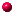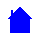## P10C - MechanicsLECTURE NOTESEquations of MotionVectorsEquations for Uniform Circular MotionMotion in two and three dimensionsForces as the cause of MotionAngles on the inclined planeEnergy, Work and the Work-Energy Theorem[NEW]Multiple forces at work on a body and Working with springs[NEW]Potential enery and conservation of energy[NEW]Impulse force changes motion in collisionsEquations derived for elastic collisionsRocket equations derivedForce of Gravity and the gravitational field strength[NEW](simple circular) Orbital Energy calculations[NEW]Smooth Rolling[NEW]Force, Torque and Angular Momentum[NEW]Use of Vector CROSS PRODUCT in Torque and Angular Momentum calculations[NEW]See this caution against dividing by unknown variables

Contact Stephen Mendes by any of the following:
Cellphones:
230-4818
266-8027
Blackberry Messenger:
21B7D748P10C Homepage